# 基本案例

HMM的主要挑战是预测隐藏部分。我们如何识别“不可观察”的事物？HMM的想法是从可观察的事物来预测潜在的事物。

# 模拟数据

library(knitr)
library(kableExtra)
library(dplyr)
theta_v <- data.frame(t(c(2.00,-2.00,1.00,2.00,0.95,0.85)))
names(theta_v) <- c("$\\mu_1$","$\\mu_2$","$\\sigma_1$","$\\sigma_2$","$p_{11}$","$p_{22}$")
kable(theta_v, "html", booktabs = F,escape = F) %>%
kable_styling(position = "center")

## 马尔可夫过程

p11 <- theta_v[1,5]
p22 <- theta_v[1,6]
P <- matrix(c(p11,1-p22,1-p11,p22),2,2)
P[1,]

##  0.95 0.05

set.seed(13)
T_end <- 10^2

s0 <- 1
st <- function(i) sample(1:2,1,prob = P[i,])

s <- st(s0)
for(t in 2:T_end) {
s <- c(s,st(s[t-1]))
}
plot(s, pch = 20,cex = 0.5)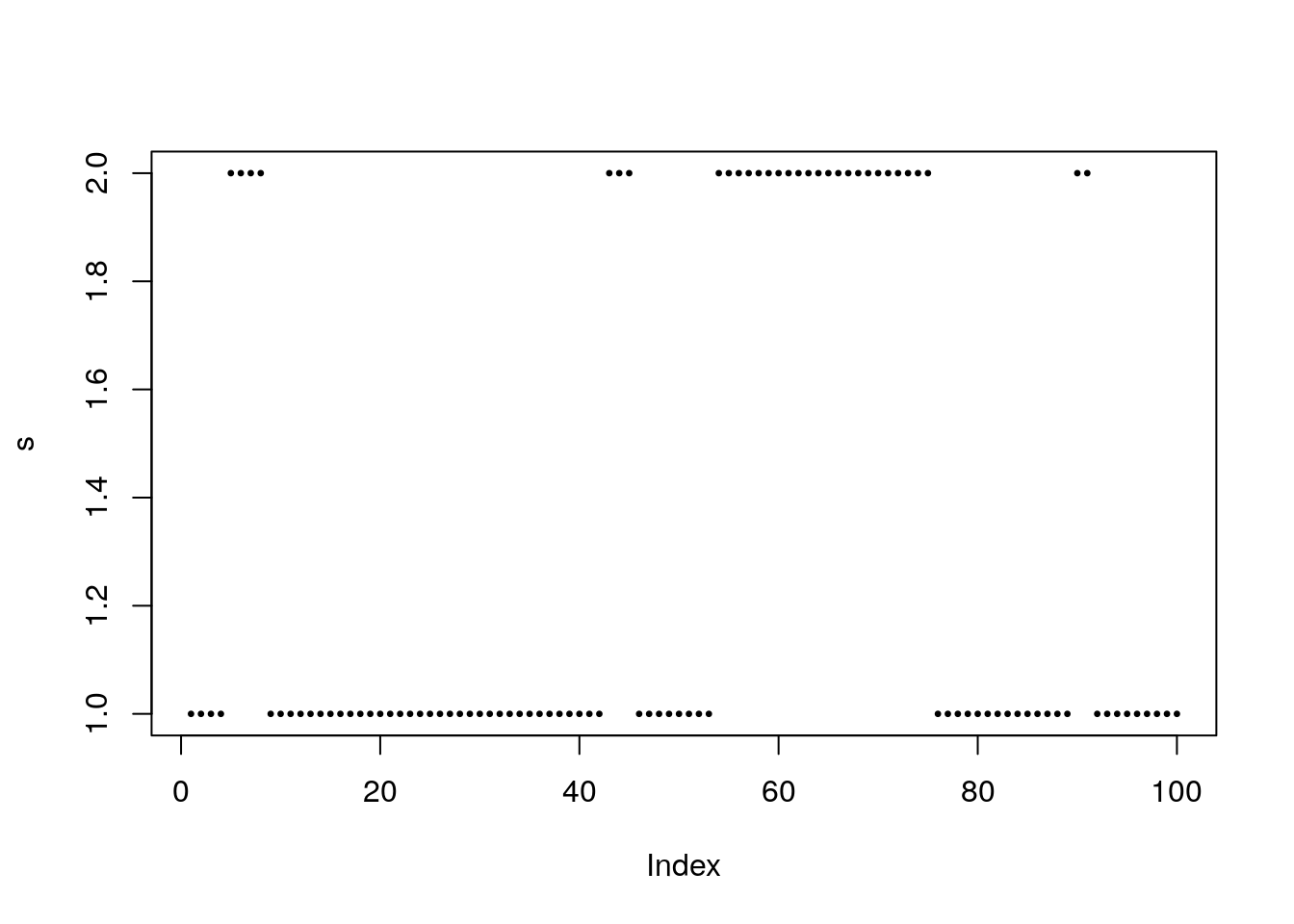P_stat[1,]

##  0.75 0.25

mean(s==1)

##  0.69

## 结果

plot(x~t_index, pch = 20)
points(x[s == 2]~t_index[s==2],col = 2)

# 在优化似然函数之前。让我们看一下工作原理。假设我们知道参数 Theta 的向量，并且我们有兴趣使用 x_t 上的数据评估随时间变化的隐藏状态。

all(round(apply(Filter[,-1],1,sum),9) == 1)

##  TRUE

plot(Filter[,3]~t_index, type = "l", ylab = expression(xi))
points(Filter[s==2,3]~t_index[s==2],pch = 20, col = 2)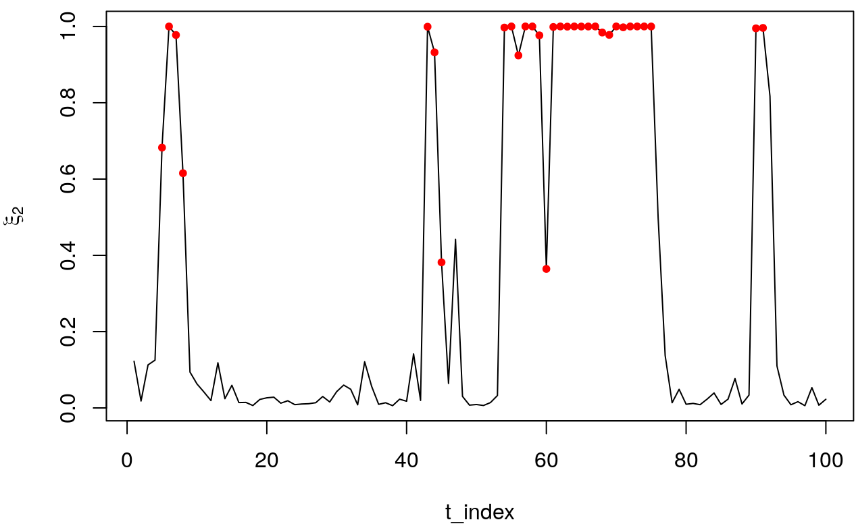### 手动估算

all(A%*%theta0 >= B)

##  TRUE

## $par ##  1.7119528 -1.9981224 0.8345350 2.2183230 0.9365507 0.8487511 ## ##$value
##  174.7445
##
## $counts ## function gradient ## 1002 NA ## ##$convergence
##  0
##
## $message ## NULL ## ##$outer.iterations
##  3
##
## $barrier.value ##  6.538295e-05 为了检查MLE值是否与真实参数一致，我们绘制估计值与真实值的关系图： plot(opt$par ~ theta_known,pch = 20,cex=2,ylab="MLE",xlab = "True")
abline(a=0,b=1,lty=2)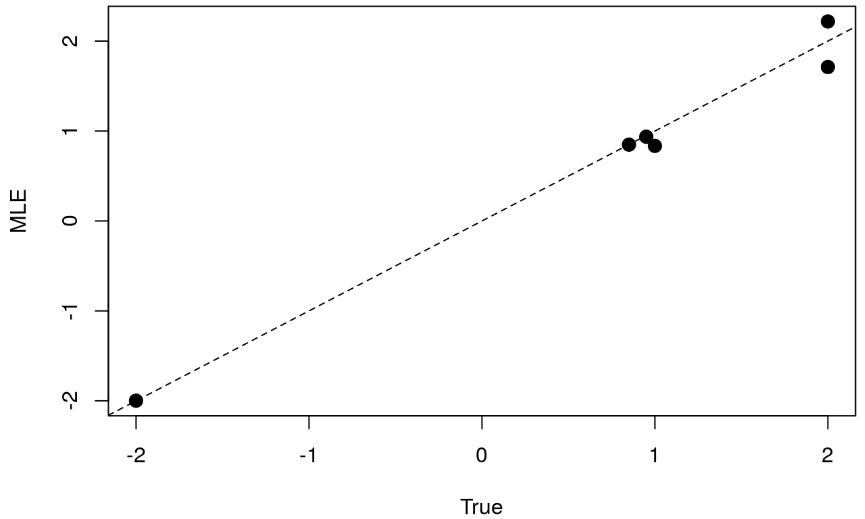### 估算

kable(mod_est, "html", booktabs = F,escape = F) %>%
kable_styling(position = "center")

EX <- 0.75*2 + 0.25*-2
EX

##  1

EX2 <- (2^2 + 1^2)*0.75 + ((-2)^2 + 2^2)*0.25
VX <- EX2 - EX^2
sqrt(VX)

##  2.179449

# 我们注意到，回归估计值与波动率的一致性高于均值。

## Markov Switching Model
##
##
##        AIC     BIC    logLik
##   352.2843 366.705 -174.1422
##
## Coefficients:
##         (Intercept)(S)    Std(S)
## Model 1       1.711693 0.8346013
## Model 2      -2.004137 2.2155742
##
## Transition probabilities:
##            Regime 1  Regime 2
## Regime 1 0.93767719 0.1510052
## Regime 2 0.06232281 0.8489948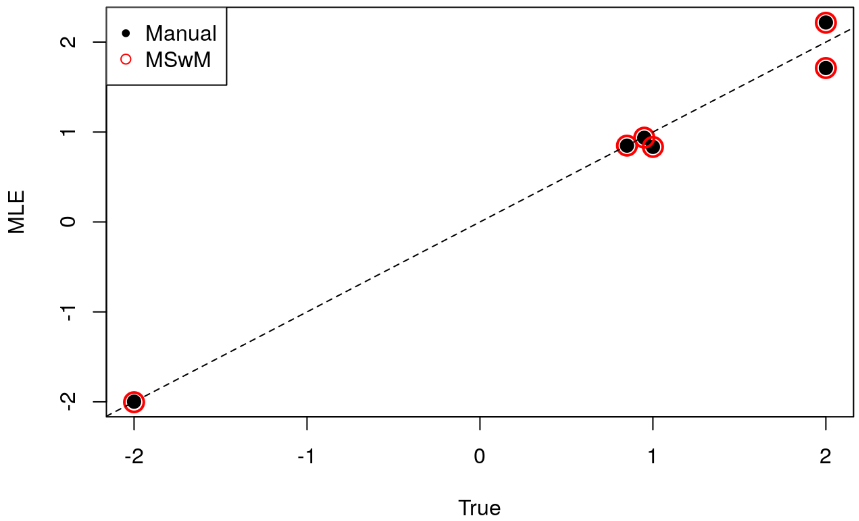par(mar = 2*c(1,1,1,1),mfrow = c(2,1))
plotProb(mod.mswm,2)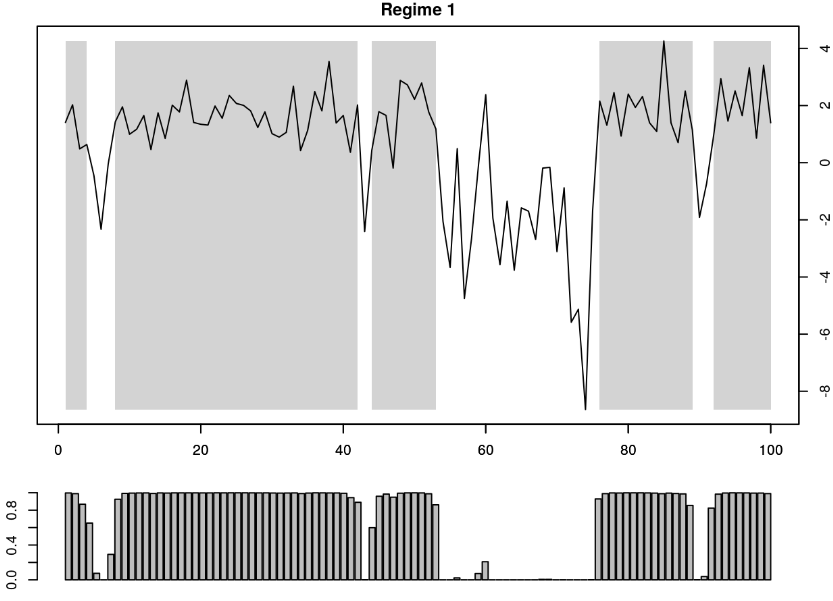plot(x~t_index,type ="l",col = 0,xlim=c(1,100))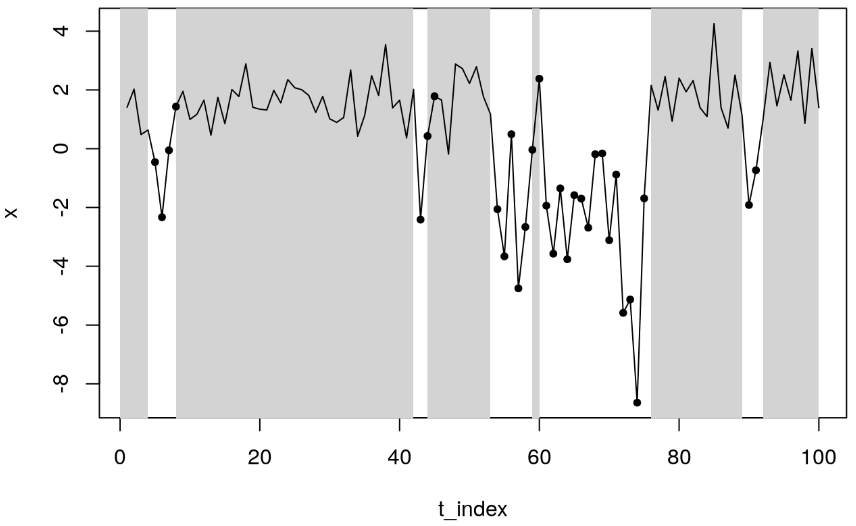mean(Filter\$Regime_1 == (s==1)*1)

##  0.96

# 结束语

Kaizong Ye拓端研究室（TRL）的研究员。

​非常感谢您阅读本文，如需帮助请联系我们！QQ在线咨询

15121130882

0571-63341498

## 关注有关新文章的微信公众号

This will close in 0 seconds